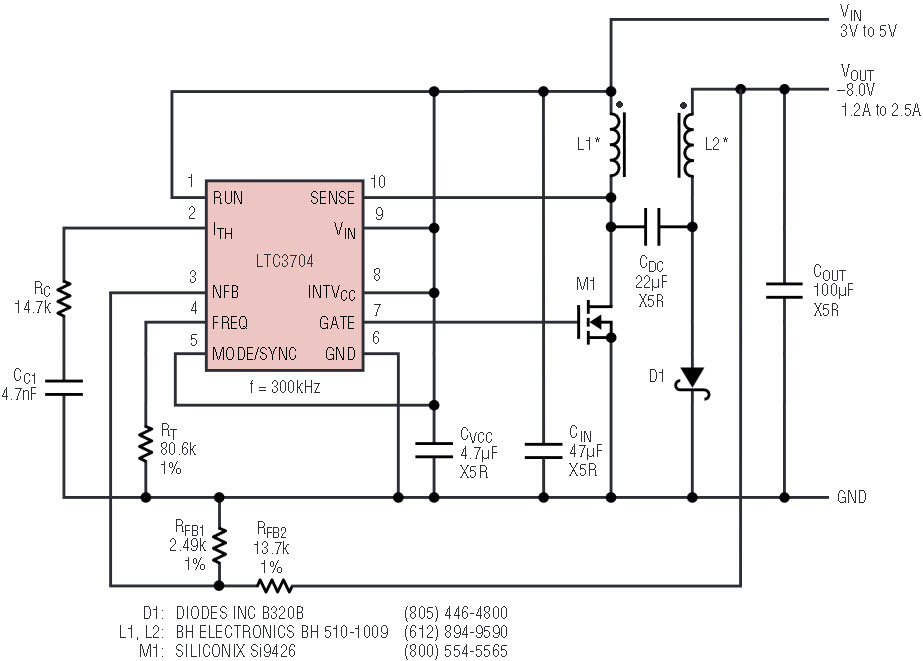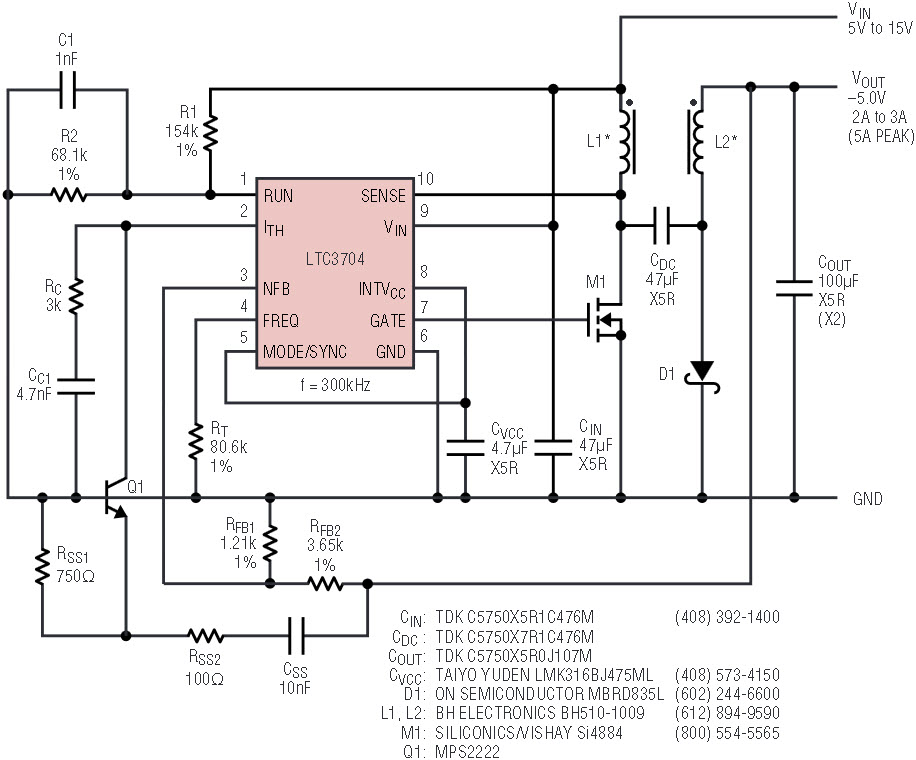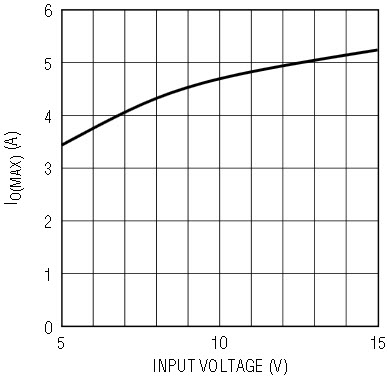# A High Performance, Single-Ended, No RSENSE Controller for Negative Output Supplies

### Introduction

The LTC3704 is a general-purpose, single-ended current-mode DC/DC controller IC that is optimized for use in negative output converter applications. Unlike traditional controllers, which are optimized for positive outputs, the LTC3704 has a negative feedback pin (NFB), which can be connected directly to a divider from the negative output to ground. This direct connection saves space and expense by eliminating the traditional glue circuitry normally required to level-shift the feedback signal above ground. The LTC3704 provides flexible, high performance operation in a small MS10 package.

The LTC3704 takes advantage of Analog Devices' proprietary No RSENSE technology by sensing the voltage across the power MOSFET, as shown in Figure 1. This sensing technique improves efficiency and reduces the size and cost of the solution. For applications where the drain of the power MOSFET exceeds 36V, or when more accurate control of the maximum current is important, the LTC3704 can also be used with a conventional sense resistor in the source of the power MOSFET.Figure 1. A 3V to 5V input, –8V output at 1.2A RF power supply.

The operating frequency of the LTC3704 can be programmed over a 50kHz to 1MHz range with a single resistor from the FREQ pin to ground. In addition, for systems where the switching frequency of the converter needs to be controlled by an external clock, the LTC3704 can be synchronized using the MODE/SYNC pin.

The LTC3704 exhibits low quiescent current and is capable of operating with an input voltage from 2.5V to 36V. When operating in Continuous Conduction Mode (CCM), the quiescent current drawn by the IC is typically only 550µA plus the current required to switch the gate of the external power MOSFET (IQTOT = 550µA + QG • fOSC). In Burst Mode® operation, at light loads, this total quiescent current (IQTOT) can drop to as low as 250µA. Furthermore, when the chip is in Shutdown Mode (the voltage at the RUN pin is below 1.248V) the total quiescent current drops to a very low 10µA.

### A High Efficiency, –8V, 1.2A RF Power Supply

Figure 1 illustrates a –8V, 1.2A (2.5A peak) RF power supply which can operate from a 3V to 5V input supply. This design takes advantage of the No RSENSE technology in order to maximize efficiency, reduce board space and reduce the overall cost of the supply. Operating at a switching frequency of 300kHz enables the use of a small, 1:1 coupled inductor. An SO-8 power MOSFET (which has a maximum RDS(ON) of 16mΩ at VGS = 2.5V and 13.5mΩ at VGS = 4.5V) and a surface mount diode provide the 1.2A to 2.5A output current level. All of the capacitors in this design are low ESR, X5R-dielectric ceramics. The output current can easily be scaled by the choice of the components around the chip without modifying the basic design.

Figure 2 shows the efficiency at 3V and 5V input, and Figure 3 shows the maximum output current vs input voltage. Figure 4 shows the load step response at 3V and 5V input.Figure 2. Efficiency vs output current for the converter in Figure 1.Figure 3. Maximum output current vs input voltage for the converter in Figure 1.Figure 4a. Load step response with 3V input for the converter in Figure 1.Figure 4b. Load step response with 5V input for the converter in Figure 1.

### A 5V to 15V Input, –5V Output at 3A (5A Peak) Converter with Soft Start and Undervoltage Lockout

The negative output converter illustrated in Figure 5 can operate with a DC supply as low as 5V. It also takes advantage of the No RSENSE technology in order to maximize efficiency. This design uses the same 1:1 coupled-inductor, along with an Si4884 power MOSFET (which has a maximum RDS(ON) of 16.5mΩ at VGS = 4.5V). The ceramic capacitor used for the DC coupling capacitor provides low ESR and high RMS current capability.Figure 5. A 5V to 15V input, –5V output at 2A positive-to-negative power supply with soft start and undervoltage lockout.

Figure 6 shows the efficiency for this converter at three input voltages, while Figure 7 shows the maximum output current vs input voltage.Figure 6. Efficiency vs output current for the converter in Figure 5.Figure 7. Maximum output current vs input voltage for the converter in Figure 5.

The soft start circuit consists of the NPN transistor Q1, combined with resistors RSS1 and RSS2, and capacitor CSS. When the circuit first starts up, the VBE of Q1, across resistor RSS1, defines the current through capacitor CSS. Since I = C • dV/dt, the dV/dt at the output is controlled by the values of RSS1 and CSS (the VBE is constant at about 0.7V). Resistor RSS2 is included to buffer the emitter of Q1 from the capacitance of CSS. Figure 8 illustrates the start-up characteristics of the supply.

Resistors R1 and R2 in Figure 5 program the input voltage turn-on and turn-off thresholds for the converter (4.4V rising and 4.1V falling, respectively). Sensing the input voltage is useful in battery applications, and to protect the power MOSFET from overheating at low gate-to-source voltages. For a falling input voltage (such as a discharging battery), the RUN pin on the LTC3704 is compared to an internal, trimmed, micropower 1.248V reference. If the RUN pin falls below this threshold, the chip shuts down and the quiescent current drops to 10µA in order to reduce the load on the battery. The 100mV hysteresis on the RUN pin comparator compensates for the rise in the unloaded battery voltage (or other input supply) and provides good noise immunity in general. Capacitor C1 provides a small amount of “ride-through” capability for short undervoltage conditions.

This design greatly benefits from a 5.2V Low Dropout Regulator (LDO) built into the LTC3704. Having such a wide input voltage range (5V to 15V) would normally present problems for the gate drive to the power MOSFET. At high input voltages, the reliability of the MOSFET is degraded dramatically. At low DC input voltages, any drop between the chip supply and the gate driver output reduces the VGS of the MOSFET, forcing the use of a sub-logic-level power MOSFET rated at a VGS of 2.5V. With a PMOS-output LDO and a strong CMOS gate driver, however, at 5V input, the full supply voltage is applied to the gate of the MOSFET with the LTC3704, providing maximum efficiency and more flexibility in the choice of power MOSFETs.

### A SLIC Power Supply

Figure 9 illustrates a multiple-output telecom power supply designed for use in Subscriber Line Interface Circuits, or SLICs. The input to the SLIC power supply is some form of battery (lead acid or lithium-ion, for example) so that talk battery power can be provided to POTS (Plain Old Telephone System) phones during an AC line failure (or rolling blackout). The output voltage is typically proportional to the distance the subscriber line runs from the local hub to a house or office, in order to compensate for the impedance of the loop. Multiple output supplies are used to supply groups of users at different distances from the hub.

The –24V output of this supply uses one secondary winding in a positive-to-negative configuration, whereas the –72V output uses the other two windings in a conventional flyback mode. The –24V output is regulated, and the –72V output is obtained by stacking additional windings on the –24V output. A standard Versapak transformer (VP5-0155) is a convenient choice, with 3 windings connected in parallel on the primary to satisfy the high current demand.

The other positive-to-negative converters described in this article have taken advantage of the No RSENSE technology, but this design cannot because it places significant voltage stress on the drain of the power MOSFET (with leakage spikes and high frequency ringing, the maximum voltage on the drain of the MOSFET can exceed 40V, and the absolute maximum rating for the SENSE pin on the LTC3704 is 36V). As a result, this design uses a 100V BVDSS device, along with a conventional 12mΩ sense resistor in the MOSFET source. The power losses incurred by this sense resistor are relatively small in this system (approximately 1%), due to the high input voltage.

A sense resistor can also improve performance in systems where the ability to control the maximum output current takes precedence over increasing overall efficiency. The initial tolerance of a discrete sense resistor is typically better than ±5%, whereas the initial tolerance of the RDS(ON) of a power MOSFET is typically ±20 to ±30%. In addition, the temperature coefficient of the discrete resistor can easily be an order of magnitude lower than for a power MOSFET (whose RDS(ON) increases approximately 50% from 25°C to 125°C).

Undervoltage conditions at the input supply are detected at the RUN pin via the resistor divider formed by R1 and R2. In this case if the battery pack discharges to below 5.0V, the converter shuts down. The rising input startup threshold is approximately 5.4V. The optional capacitor CR could be used to give the converter some ride-through capability for brief input undervoltage conditions.

### Conclusion

The LTC3704 is a versatile control IC optimized for negative voltage supplies, but useful in a wide variety of single-ended DC/DC converter topologies. It is a flexible, high performance converter in a small, convenient MS10 package. It improves efficiency, reduces the size and weight of power supplies, and saves total component and manufacturing expense. Its range of applications extends from single-cell, lithium-ion powered systems to high voltage, high power telecommunications equipment.